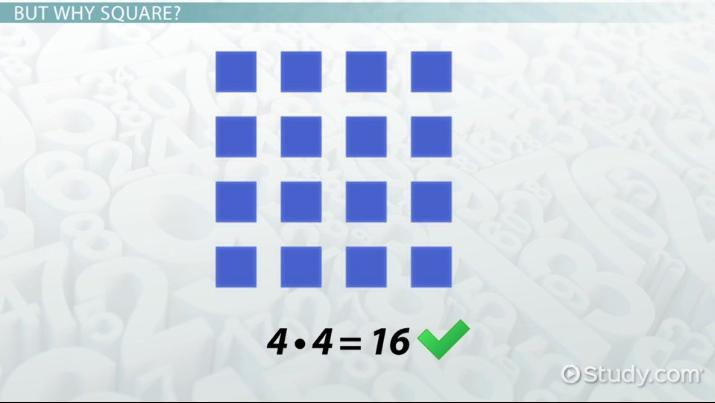# What is a Square Number in Math? - Definition & Examples

Lesson Transcript
Instructor: Joseph Vigil

Joseph has a master's degree in literature as well as alternative teaching and ESL educator certifications. He has worked with middle school, high school, and college students in writing and language arts.

In math, square numbers refer to the product of multiplying any integer by itself. Learn the complete definition of square numbers and why they are called 'square.' Calculate the given examples and find the square numbers! Updated: 10/12/2021

## Square Numbers

So, what's so special about these square numbers? What makes 1, 4, and 9, for example, square? For a simple definition, square numbers are the results you get when multiplying any integer by itself. For example, let's multiply 1 by itself:

• 1 * 1 = 1

So, one is a square number. Simple enough, right? How about the next integer: 2?

• 2 * 2 = 4

So, four is the next square number. I'll bet you can probably guess how to find the next square number. That's right! Let's multiply 3 by itself:

• 3 * 3 = 9

And so on. . .An error occurred trying to load this video.

Try refreshing the page, or contact customer support.

Coming up next: What is an Ordinal Number? - Definition & Example

### You're on a roll. Keep up the good work!

Replay
Your next lesson will play in 10 seconds
• 0:01 Square Numbers
• 0:40 But Why Square?
• 1:18 Finding Square Numbers
• 2:10 Lesson Summary
Save Save

Want to watch this again later?

Timeline
Autoplay
Autoplay
Speed Speed

## But Why Square?

Okay, that all makes sense. But, why do we call these numbers 'square?' Well, let's make a diagram of the previous multiplication sequence. If we're multiplying 3 by 3, that means we have 3 groups of 3 items.

As you can see, we have a grid measuring three items vertically and three items horizontally, and these items, when arranged in this way, make a square. We can add another row of items going both ways. The resulting diagram would look like this. We now have a square of 4 items vertically and 4 items horizontally, which gives us a total of 16 items (a square number).

## Finding Square Numbers

So, now you have the tools to find every square number in existence. Just multiply any integer by itself, and the result is a square number. And, it's called a square number because we can make a diagram of that many items, and it will take the shape of a square.

But, how would this play out in the classroom? Let's say your teacher asks, 'What is 4 squared?' This is just another way of asking, 'What do we get when we multiply 4 by 4?' The result is 16.

To unlock this lesson you must be a Study.com Member.

### Register to view this lesson

Are you a student or a teacher?